## Example Questions

### Example Question #1 : Complex Fractions

Simplify: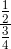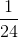Explanation:

Rewrite this complex fraction using a division sign.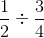Take the reciprocal of the second term and change the division of the division sign.  Simplify.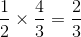### Example Question #2 : Complex Fractions

Solve: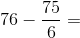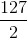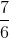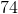Explanation:

First reduce the fraction. We can divide both the numerator and the denominator by 3.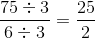Now our expression looks like this: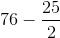When you add or subtract fractions, you need to have the same denominator. The lowest common deonminator here is 2. So we need to multiply and solve: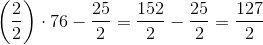### Example Question #3 : Complex Fractions

Add: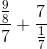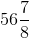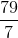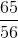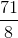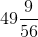Explanation:

To add, first simply each term by rewriting the terms using a division sign.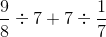Take the reciprocal of the terms after the division sign, and change the division sign to a multiplication sign. Simplify.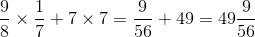### Example Question #4 : Complex Fractions

Add: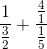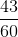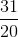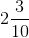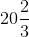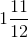Explanation:

The terms shown are complex fractions.  We first need to simplify each and find the least common denominator before solving.

Rewrite the complex fraction using a division sign.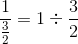Change the division sign to a multiplication sign, and take the reciprocal of the second term.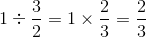Repeat this step for the second term.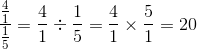The answer is: